# Introduction

In this vignette, we focus on the different visualizations you can generate by changing the arguments for the image and barplot methods. The main arguments to image, when using a CytobandData object from the package are

• what: A list or vector indicating which data columns to show.
• chr: The chromosome to plot, which can take on the value all to show the whole genome

The barplot method only takes the what argument, which in this case must be a length-one character vector.

To produce the figures in this gallery, we will use a dataset that comes with the package, already processed,

``````library(RCytoGPS)
data(cytoData)
colnames(cytoData)``````
``````##   "Chromosome" "loc.start"  "loc.end"    "Band"       "Arm"
##   "A.Loss"     "A.Gain"     "A.Fusion"   "B.Loss"     "B.Gain"
##  "B.Fusion"   "C.Loss"     "C.Gain"     "C.Fusion"``````
``````bandData <- CytobandData(cytoData)
Acolumns <- c("A.Loss", "A.Gain", "A.Fusion")``````

The banddata object includes data at the resolution of a cytoband for three sets of samples (denoted A, B, and C), describing the frequency of loss, gain, and fusion events for each set.

# Plotting Cytoband Data Along the Genome

The first visual is a barplot to show the frequencies of cytogenetic events mapped to the associated chromosome. The main parameters for `barplot` are (1) the CytobandData object containing the dataset, (2) the specific data column that you want to graph, and (3) the color of the graph.

``````opar <- par(mfrow=c(2,1))
barplot(bandData, "A.Loss", col = "forestgreen")
barplot(bandData, "B.Loss", col="orange")``````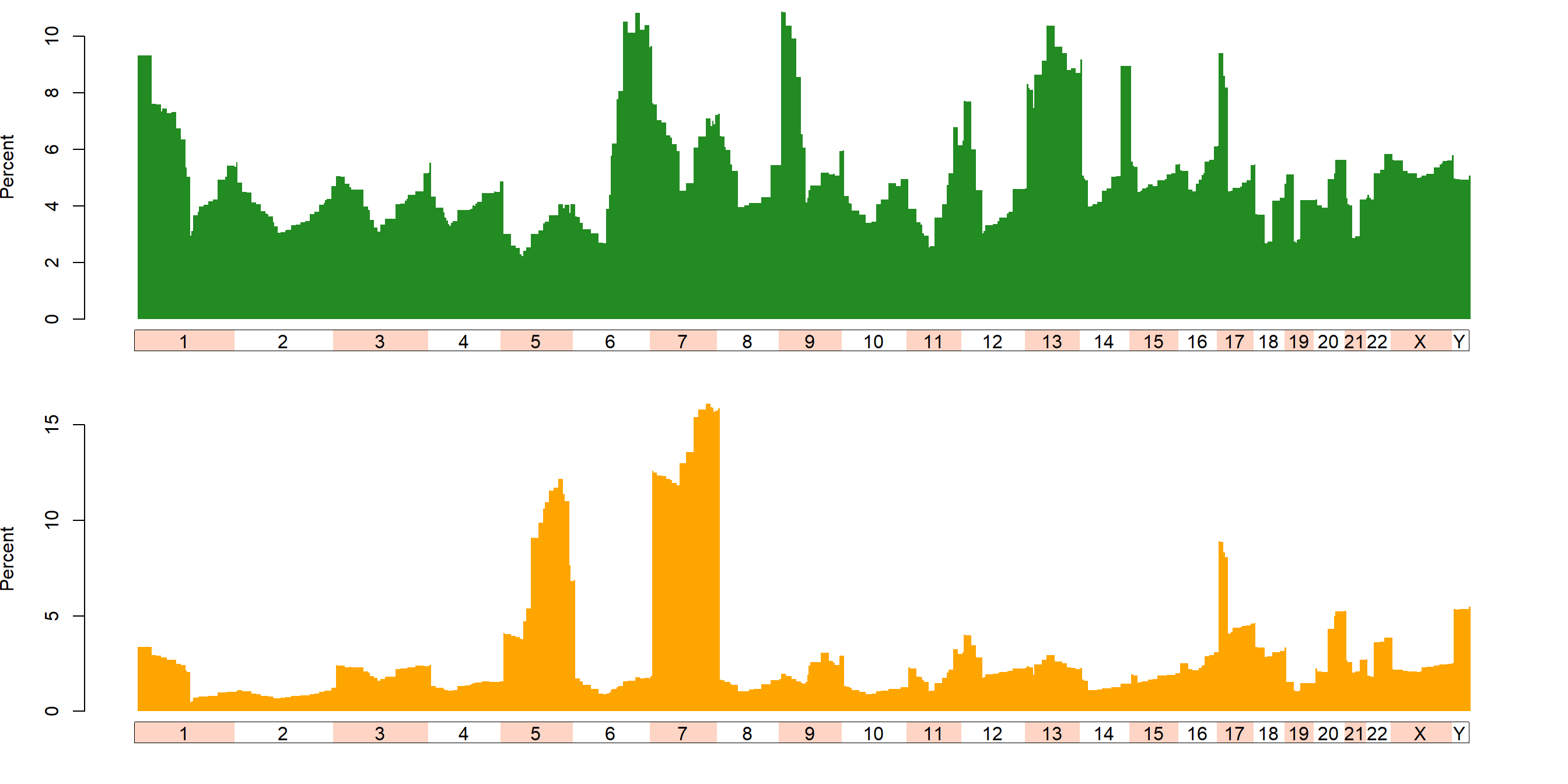Figure 1: Cytoband level data along the genome.

``par(opar)``

# Single Chromosome Plots

Plots of the data along one chromosome can be oriented either horizontally or vertically. By (our) definition, “horizontal” and “vertical” refer to the orientation of the chromosome with its cytoband shading. Unless specified, the horiz argument is set to FALSE by default.

## Plotting Cytoband-Level Data Along One Chromosome

The “vertical stacked chromosome” allows you to see a vertical barplot and its associated chromosome on the y axis, this allows you to see multiple cytogenetic events on a specified chromosome. The parameters to produce this image are the dataset, the cytogenetic event, and a specific chromosome.

``image(bandData, what = Acolumns[1:3], chr = 2)``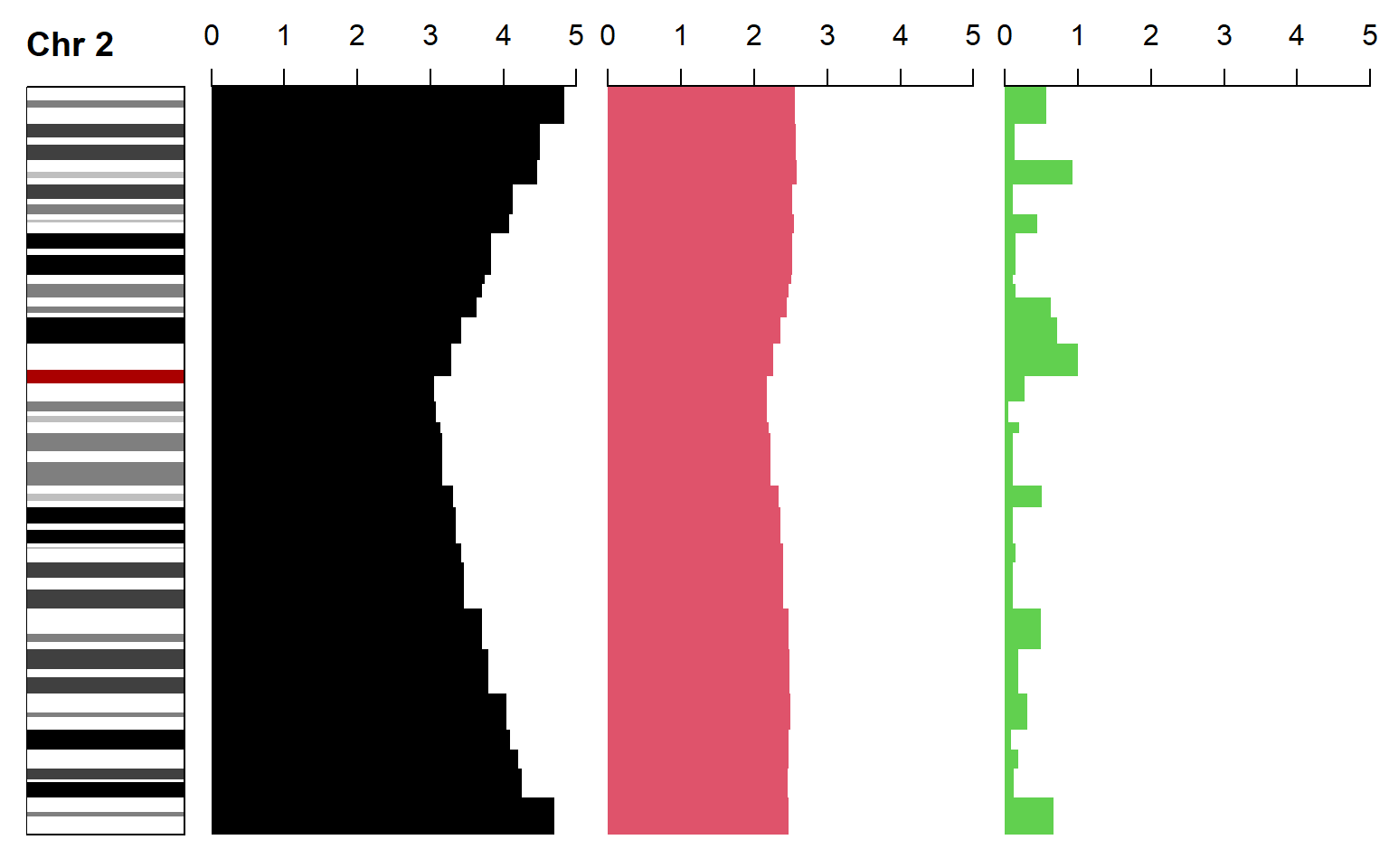Figure 2: Vertical stacked barplot of LGF frequencies on chromosome 2 for type A samples.

The next visual is nearly identical to “vertical stack chromosome” with the exception that the visual is set along the x-axis instead of the y axis.

``image(bandData, Acolumns[1:3], chr = 2, horiz = TRUE)``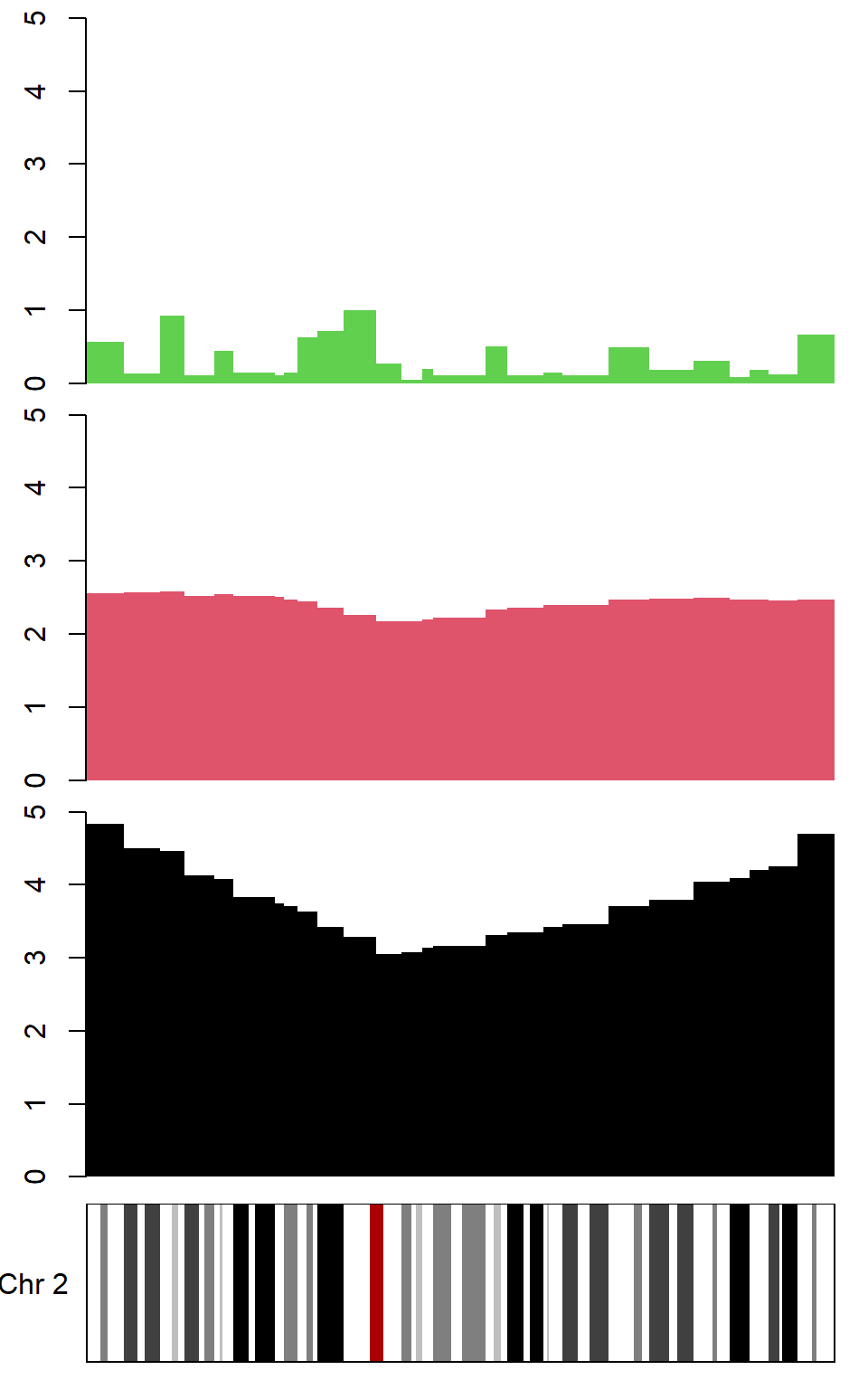Figure 3: Horizontal stacked barplot of LGF frequencies on chromosome 2 for type A samples.

## Plotting Cytoband-Level Data Along Both Sides of One Chromosome

This set of visuals allows the user to directly compare two sets of cytogenetic events on a specified chromosome on the y-axis.

``image(bandData, what = list("A.Loss", "B.Loss"), chr = 5)``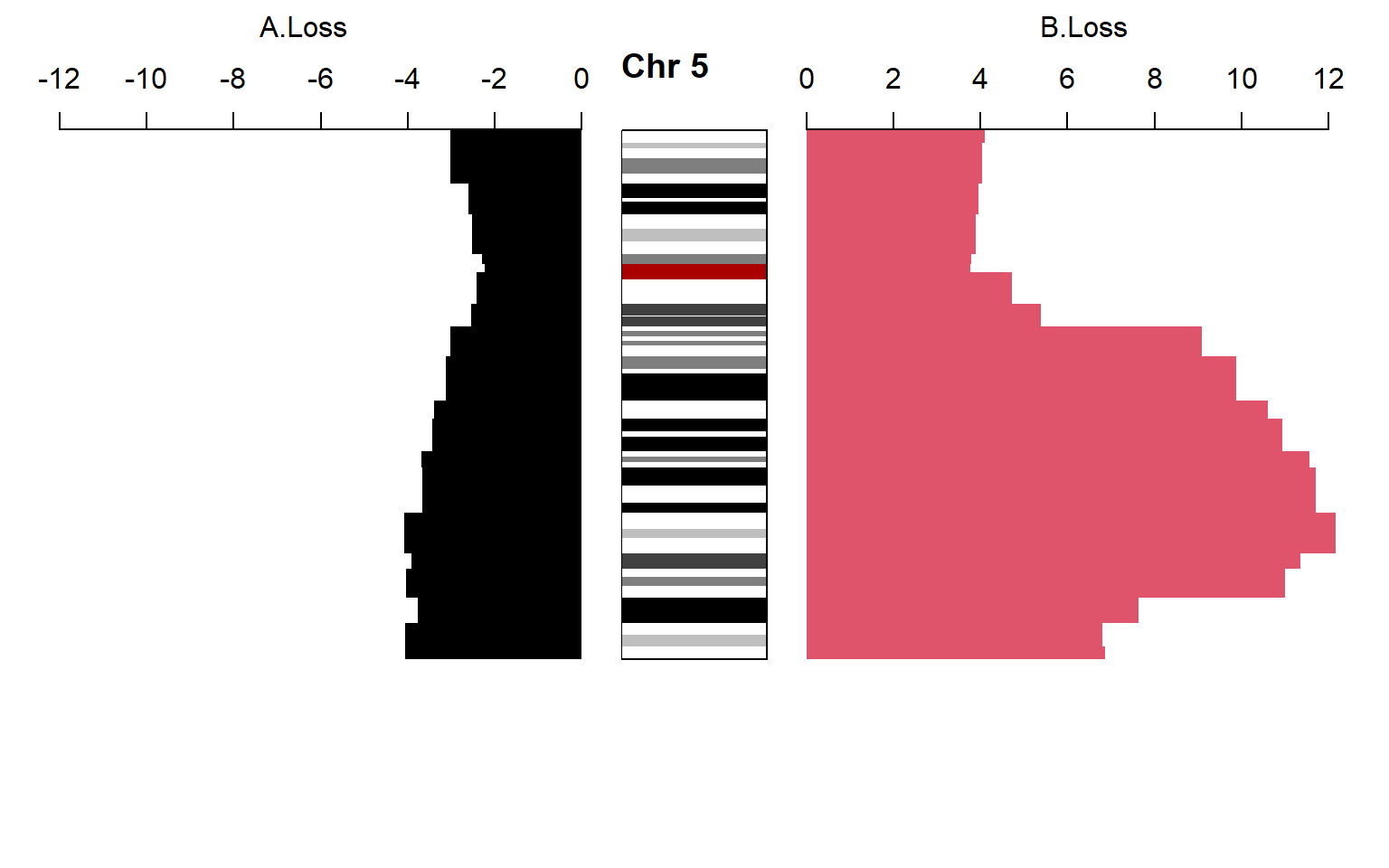Figure 4: Vertical stacked barplot of LGF frequencies on chromosome 5 for type A and B samples.

The next visual is similar to the previous visual except that the orientation is switched from vertical to horizontal.

``image(bandData, what = list("A.Loss", "B.Loss"), chr = 5, horiz = TRUE)``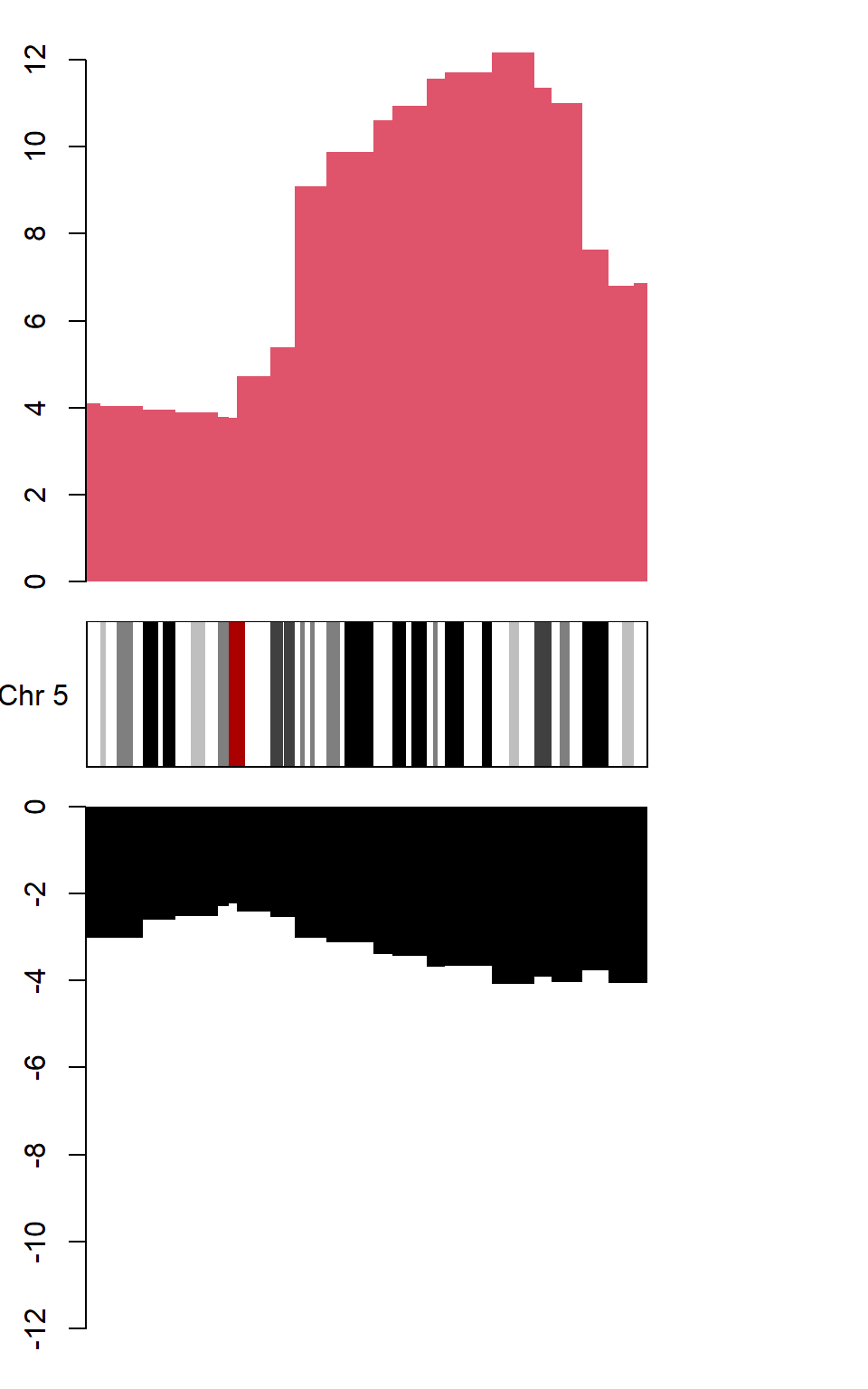Figure 5: Horizontal stacked barplot of LGF frequencies on chromosome 5 for type A and B samples.

# Idiograms

We can also produce “idiograms” that show the data for all chromosomes at once rather than just one chromosome at a time.

## One Data Column

The simplest idiogram visualizes a single data colum of cytogenetic events for all chromosomes. The parameters are similar to the previous visuals with the exception that chr is set to `all` and we describe the colors using the argument pal (for pallete).

``image(bandData, what = Acolumns, chr = "all", pal = "orange")``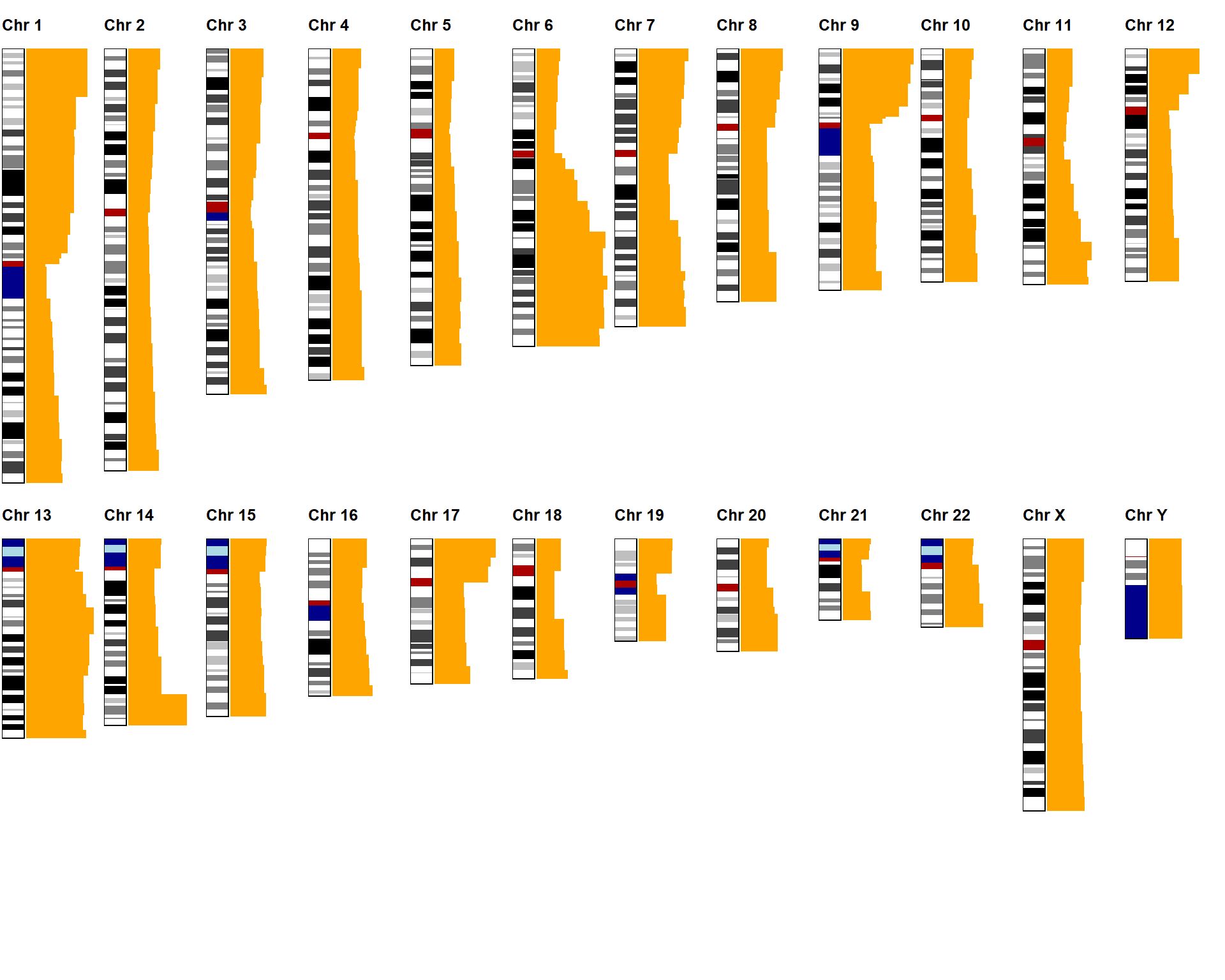Figure 6: Idiogram for one data columm.

## Contrasting Two Data Columns

This image allows you to do a comparison between two cytogenetic events on all the chromosomes.

``````image(bandData, what = Acolumns[1:2], chr = "all",
pal=c("orange", "forestgreen"))``````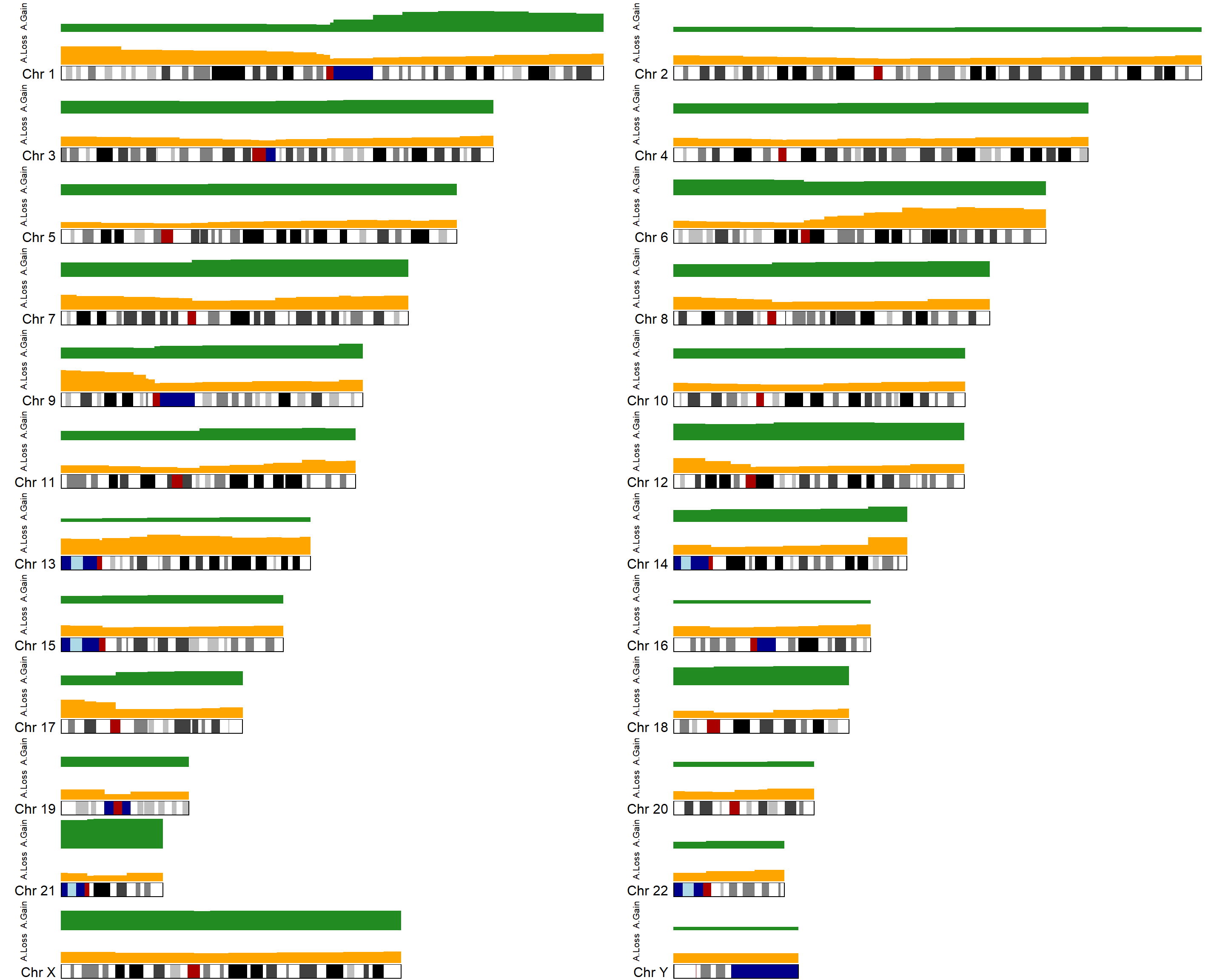Figure 7: Idiogram to contrast two data columms.

The next visual is the same as the previous with the exception that the user can dictate how many total rows they want to display. (The default is 2; permitted values range from 1 to 4.)

``````image(bandData, what = Acolumns[1:2], chr = "all", nrows = 3,
pal=c("orange", "forestgreen"))``````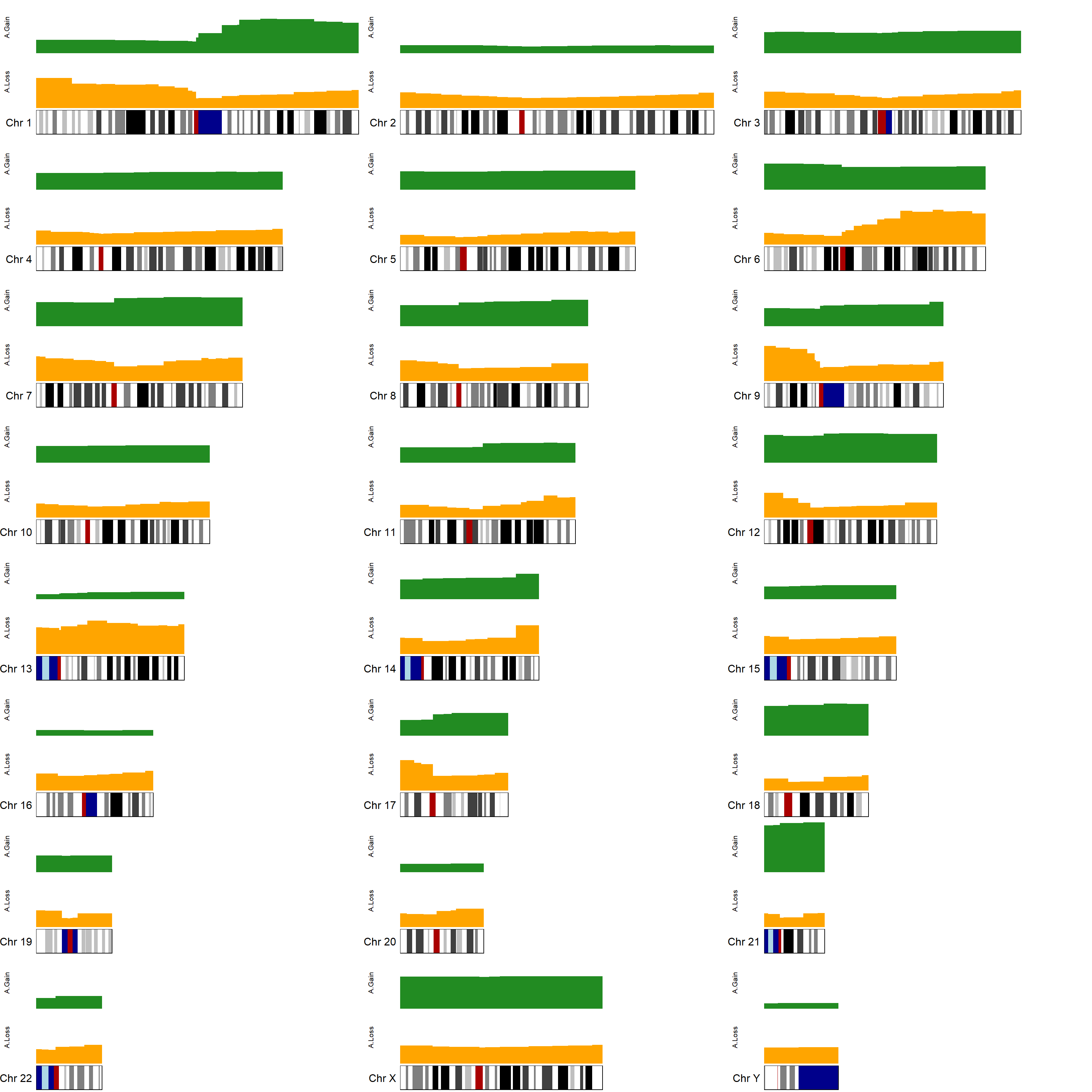Figure 8: Idiogram to contrast two data columms.

This visual is the same as before except that we have switched from vertical to horizontal.

``````image(bandData, what = Acolumns[1:2], chr = "all",
pal=c("orange", "forestgreen"), horiz = TRUE)``````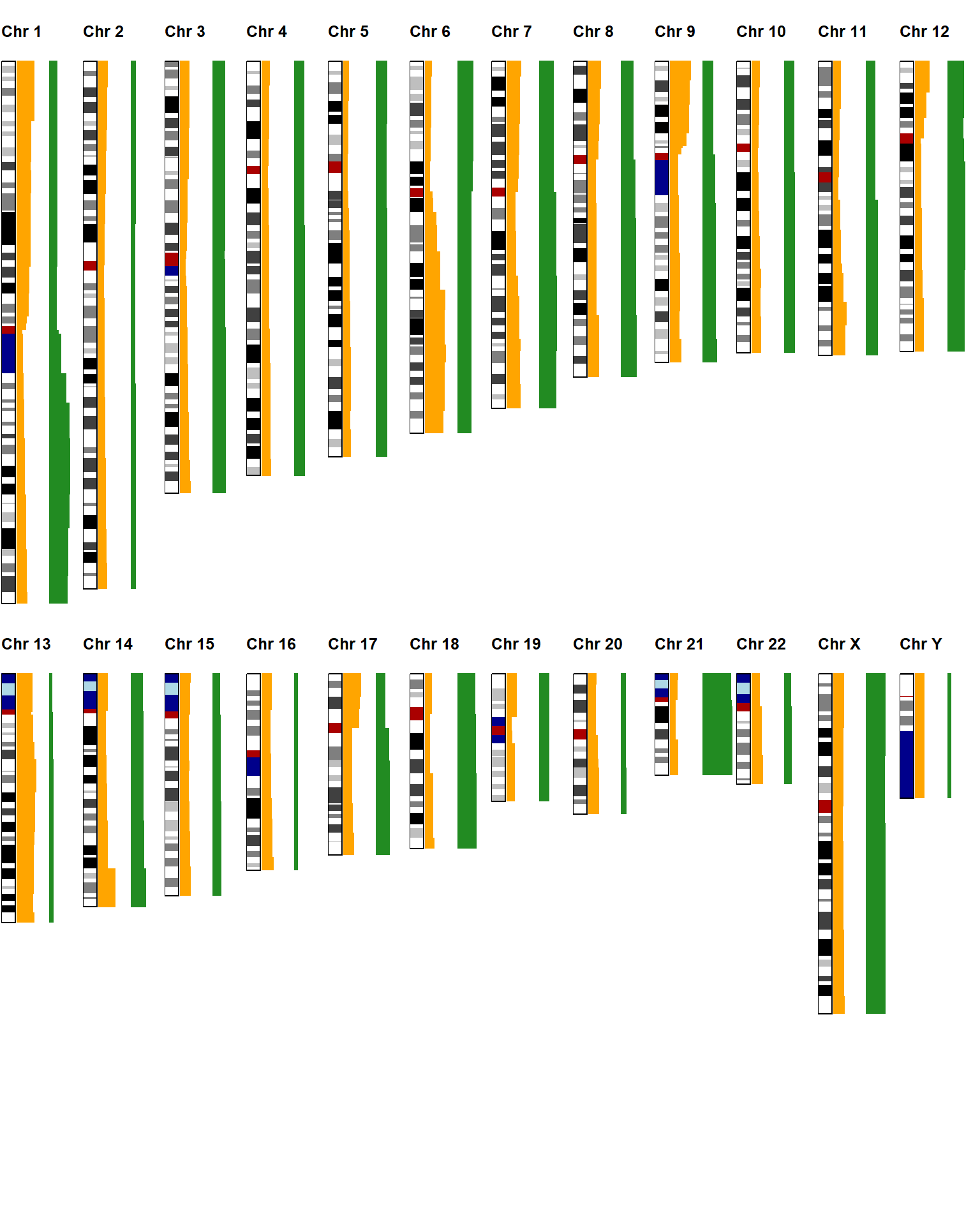Figure 9: Idiogram to contrast two data columms.

## Many Data Columns

The image method also allows you to graph more than two (up to ten) data columns.

``````image(bandData, what = Acolumns[1:3], chr = "all",
pal=c("forestgreen", "orange", "purple"), nrows=3, horiz = FALSE)``````